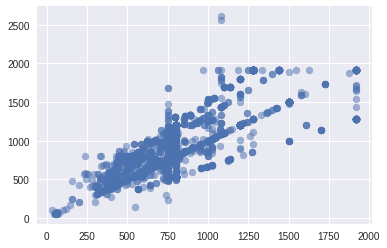# [lesson2] Accuracy Rate Less Than 20% with Resnet34

Hello world,

I am currently using resnet34 to train on my own data set. The data are dress images with around 200 classes. The distribution of the image size are as follows (x-axis is row size, y-axis is column size):I followed the steps in lesson 2 to train the model. It turns out that the accuracy rate has only around 10% accuracy.

With such initial performance, is it possible to boost accuracy up to 70% with resnet34 architecture? Or should I consider other architecture such as resnext50 or resnext101?

*Attached are an extract of my code for your reference of my model and data spec:

%matplotlib inline

import sys
sys.path.append(’/home/r8user2/fastai/old’)
from fastai.imports import *
from fastai.transforms import *
from fastai.conv_learner import *
from fastai.model import *
from fastai.dataset import *
from fastai.sgdr import *
from fastai.plots import *
import matplotlib.pyplot as plt
PATH = ‘/home/r8user2/Documents/HY/dress_data/datasets’
sz = 224
arch = resnet34
data = ImageClassifierData.from_paths(path = PATH,
tfms = tfms_from_model(arch, sz),
trn_name = ‘imgtrain’,
val_name = ‘imgval’,
bs = 64,
# utilize CPUs in remote
num_workers = 60)
learn = ConvLearner.pretrained(arch, data, precompute = True)
learn.fit(0.01, 20)

At the end of the training, it yields accuracy rate = 14.4% (training loss = 4.22, validation = 4.04)